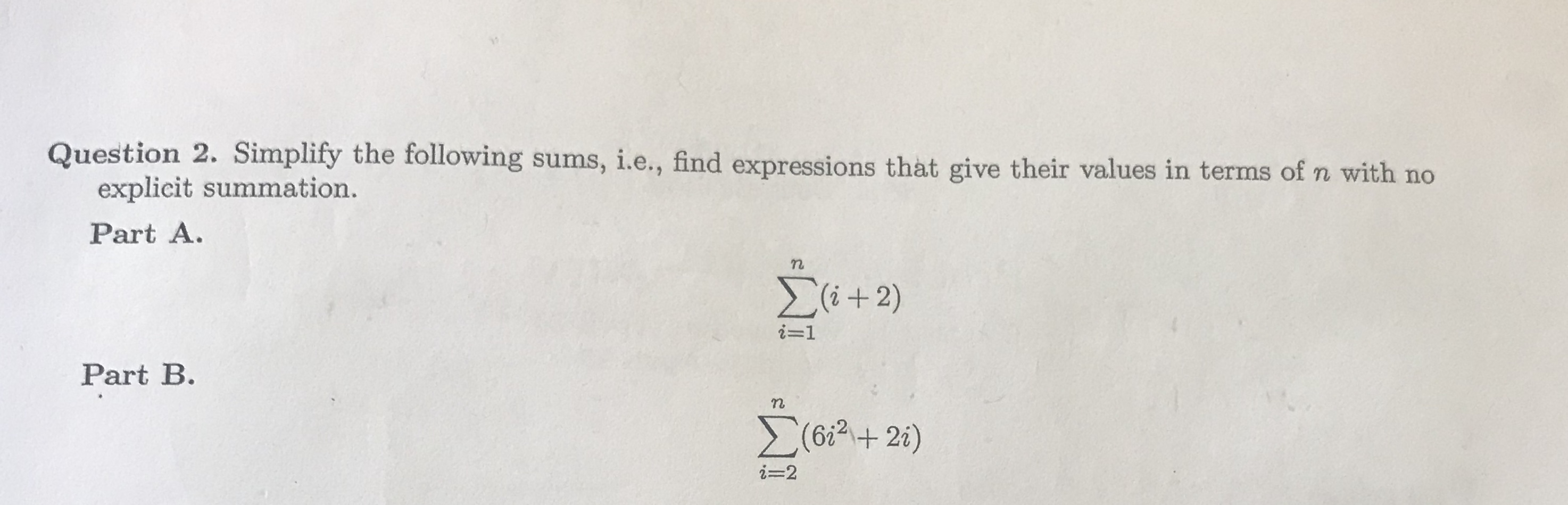# Question 2. Simplity the following sums, i.e, find expressions that give their values in terms of n with no, i.e., find expressions that give their values in terms of n with noexplicit summation.Part A.ー1Part B.6i2+ 2i-2

Question
71 views

How would you go about solving number 2help_outlineImage TranscriptioncloseQuestion 2. Simplity the following sums, i.e, find expressions that give their values in terms of n with no , i.e., find expressions that give their values in terms of n with no explicit summation. Part A. ー1 Part B. 6i2+ 2 i-2 fullscreen
check_circle

Step 1

To simplify the provided sum that is calculate the expression in term of n with no explicit summation. Now, first solve the Part A. then solve the Part B. by changing the limit of summation and the provided sums is shown below,

Step 2

First consider the sum in Part A. which is shown below,

Step 3

Further simplify the ab...

### Want to see the full answer?

See Solution

#### Want to see this answer and more?

Solutions are written by subject experts who are available 24/7. Questions are typically answered within 1 hour.*

See Solution
*Response times may vary by subject and question.
Tagged in

### Calculus